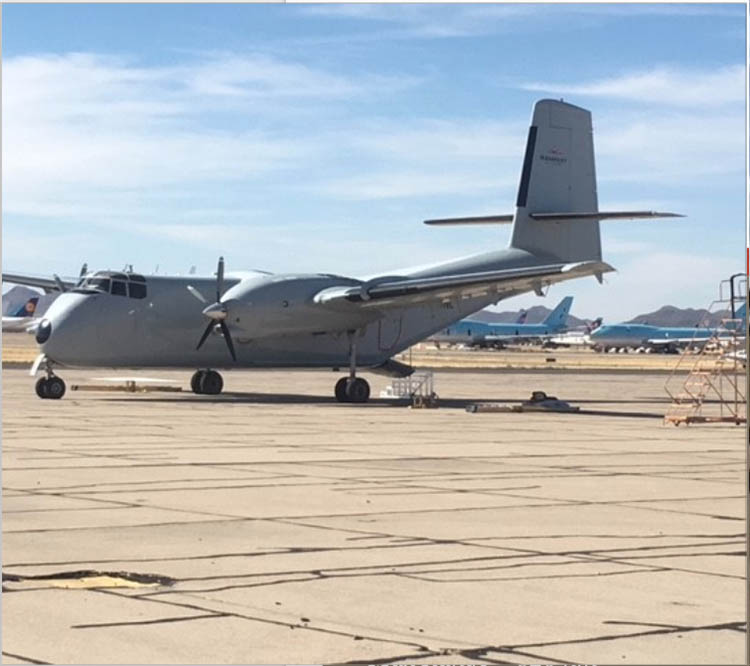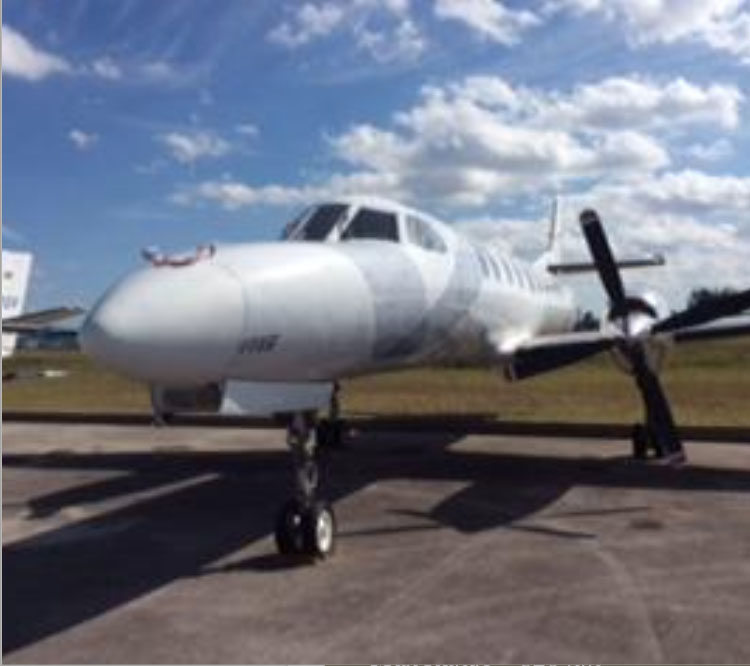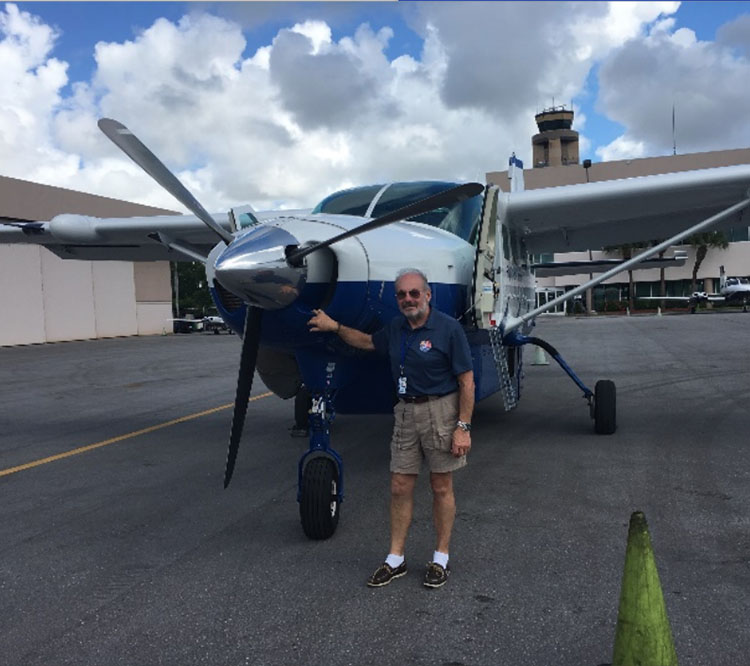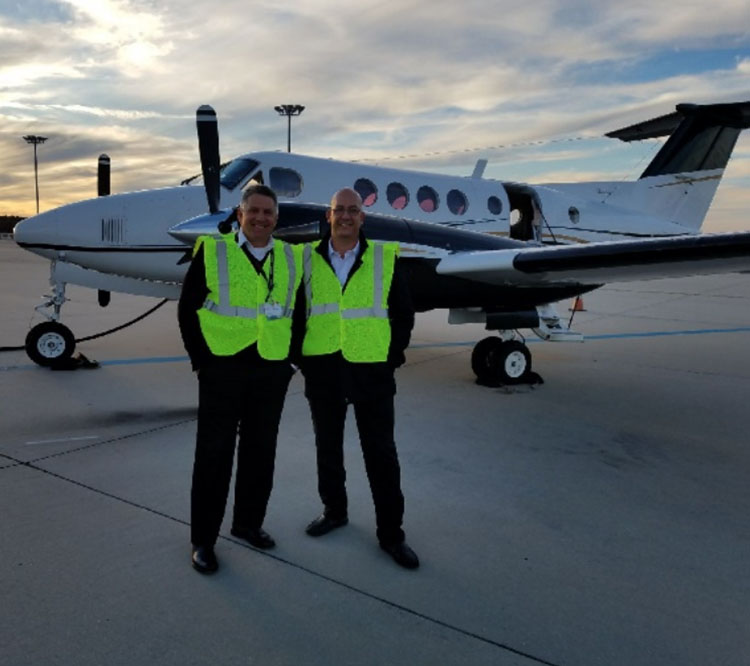CARIBOU DHC-4A

(2) PT6A Efficient Turbo Prop Engines
Cabin Volume = 1150 CU. Feet
Usable Weight = 12,500 Lbs.
Max Range = 820 nm
Fuel Burn = 150 us gal per hour
Cruising Speed = 185 mphFairchild Metro III

(2) Garrett TPE 331 Efficient
Turbo Prop Engine
Cabin Volume = 626 CU. Feet
Usable Weight = 4400 Lbs.
Max Range = 1150 nm
Fuel Burn = 136 us gal per hour
Cruising Speed = 310 mphGrand Caravan 208

Volume = 453 CU. FEET
Usable Weight = 2000 Lbs. Plus
Max Range = 964 NM
Fuel Burn = 60 us gal per hour
Cruising Speed = 180 mphKing Air 200

Volume = 303 CU. FEET
Usable Weight = 1500 Lbs. Plus
Max Range = 1,720 NM
Fuel Burn = 132 us gal per hour
Cruising Speed = 245 mph